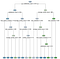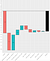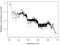Decision Tree (AUC: 0.823)A decision tree to predict employee attrition. The prediction is the label on each leaf node (eg 0.59 means 59% chance of leaving)
```Satisfaction Level = 0.23
Average Monthly Hours = 200
Last Evaluation = 0.5```
```0.24 ::: Baseline
+0.28 ::: Satisfaction Level (prediction is now 0.52)
-0.09 ::: Average Monthly Hours (prediction is now 0.43)
-0.12 ::: Last Evaluation (prediction is now 0.31)= 0.31 ::: Prediction```

The problem is that decision trees are generally not that great in terms of predictive power.

The XGBoost ExplainerThe explanation of the log-odds prediction of -1.299 (y-axis shows the probability, the bar labels show the log-odds impact of each variable)
```-1.41 ::: Baseline (Intercept)
-1.10 ::: Satisfaction Level (prediction is now -2.51)
+0.98 ::: Last Evaluation (prediction is now -1.53)
+0.32 ::: Time Spent At Company (prediction is now - 1.21)
+0.27 ::: Hours Average Monthly (prediction is now -0.94)
-0.24 ::: Sales (prediction is now -1.18)
-0.18 ::: Number of Projects (prediction is now -1.36)
+0.11 ::: Work Accident (prediction is now -1.25)
-0.07 ::: Salary (prediction is now -1.32)
+0.02 ::: Promotion Last 5 Years (prediction is now -1.30)= -1.299 ::: Prediction```

How does it work?Each point is one employee from the test set. The satisfaction level of the employee is plotted on the x-axis; the impact of the satisfaction level on the log-odds of leaving is plotted on the y-axis.

What's incredible is that we can draw this insight directly from the xgboost model, using the xgboostExplainer.

How can I get it?

```install.packages("devtools")
library(devtools)
install_github("AppliedDataSciencePartners/xgboostExplainer")```

Code for the above examples

```library(data.table)
library(rpart)
library(rpart.plot)
library(caret)
library(xgboost)
library(pROC)set.seed(123)full = fread('./data/HR.csv', stringsAsFactors = T)
full = full[sample(.N)]#### Add Random Noisetmp_std = sd(full[,satisfaction_level])
full[,satisfaction_level:=satisfaction_level + runif(.N,-tmp_std,tmp_std)]
full[,satisfaction_level:=satisfaction_level - min(satisfaction_level)]
full[,satisfaction_level:=satisfaction_level / max(satisfaction_level)]tmp_std = sd(full[,last_evaluation])
full[,last_evaluation:=last_evaluation + runif(.N,-tmp_std,tmp_std) ]
full[,last_evaluation:=last_evaluation - min(last_evaluation)]
full[,last_evaluation:=last_evaluation / max(last_evaluation)]tmp_min = min(full[,number_project])
tmp_std = sd(full[,number_project])
full[,number_project:=number_project + sample(-ceiling(tmp_std):ceiling(tmp_std),.N, replace=T)]
full[,number_project:=number_project - min(number_project) + tmp_min]tmp_min = min(full[,average_montly_hours])
tmp_std = sd(full[,average_montly_hours])
full[,average_montly_hours:=average_montly_hours + sample(-ceiling(tmp_std):ceiling(tmp_std),.N, replace=T)]
full[,average_montly_hours:=average_montly_hours - min(average_montly_hours) + tmp_min]tmp_min = min(full[,time_spend_company])
tmp_std = sd(full[,time_spend_company])
full[,time_spend_company:=time_spend_company + sample(-ceiling(tmp_std):ceiling(tmp_std),.N, replace=T)]
full[,time_spend_company:=time_spend_company - min(time_spend_company) + tmp_min]tmp_min = min(full[,number_project])
tmp_std = sd(full[,number_project])
full[,number_project:=number_project + sample(-ceiling(tmp_std):ceiling(tmp_std),.N, replace=T)]
full[,number_project:=number_project - min(number_project) + tmp_min]#### Create Train / Test and Foldstrain = full[1:12000]
test = full[12001:14999]cv <- createFolds(train[,left], k = 10)
# Control
ctrl <- trainControl(method = "cv",index = cv)#### Train Treetree.cv <- train(x = train[,-"left"], y = as.factor(train[,left]), method = "rpart2", tuneLength = 7,
trControl = ctrl, control = rpart.control())tree.model = tree.cv\$finalModel
rpart.plot(tree.model, type = 2,extra =  7,fallen.leaves = T)
rpart.plot(tree.model, type = 2,extra =  2,fallen.leaves = T)tree.preds = predict(tree.model, test)[,2]
tree.roc_obj <- roc(test[,left], tree.preds)cat("Tree AUC ", auc(tree.roc_obj))#### Train XGBoostxgb.train.data = xgb.DMatrix(data.matrix(train[,-'left']), label = train[,left], missing = NA)param <- list(objective = "binary:logistic", base_score = 0.5)
xgboost.cv = xgb.cv(param=param, data = xgb.train.data, folds = cv, nrounds = 1500, early_stopping_rounds = 100, metrics='auc')
best_iteration = xgboost.cv\$best_iterationxgb.model <- xgboost(param =param,  data = xgb.train.data, nrounds=best_iteration)xgb.test.data = xgb.DMatrix(data.matrix(test[,-'left']), missing = NA)
xgb.preds = predict(xgb.model, xgb.test.data)
xgb.roc_obj <- roc(test[,left], xgb.preds)cat("Tree AUC ", auc(tree.roc_obj))
cat("XGB AUC ", auc(xgb.roc_obj))#### Xgb importance
col_names = attr(xgb.train.data, ".Dimnames")[]imp = xgb.importance(col_names, xgb.model)
xgb.plot.importance(imp)#### THE XGBoost Explainerlibrary(xgboostExplainer)explainer = buildExplainer(xgb.model,xgb.train.data, type="binary", base_score = 0.5, trees_idx = NULL)
pred.breakdown = explainPredictions(xgb.model, explainer, xgb.test.data)cat('Breakdown Complete','\n')
weights = rowSums(pred.breakdown)
pred.xgb = 1/(1+exp(-weights))
cat(max(xgb.preds-pred.xgb),'\n')idx_to_get = as.integer(802)
test[idx_to_get,-"left"]
showWaterfall(xgb.model, explainer, xgb.test.data, data.matrix(test[,-'left']) ,idx_to_get, type = "binary")####### IMPACT AGAINST VARIABLE VALUE
plot(test[,satisfaction_level], pred.breakdown[,satisfaction_level], cex=0.4, pch=16, xlab = "Satisfaction Level", ylab = "Satisfaction Level impact on log-odds")plot(test[,last_evaluation], pred.breakdown[,last_evaluation], cex=0.4, pch=16, xlab = "Last evaluation", ylab = "Last evaluation impact on log-odds")cr <- colorRamp(c("blue", "red"))
plot(test[,last_evaluation], pred.breakdown[,last_evaluation], col = rgb(cr(round(test[,satisfaction_level])), max=255), cex=0.4, pch=16, xlab = "Last evaluation", ylab = "Last evaluation impact on log-odds")```

1.9K claps

Written by